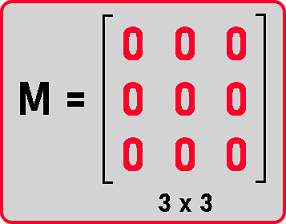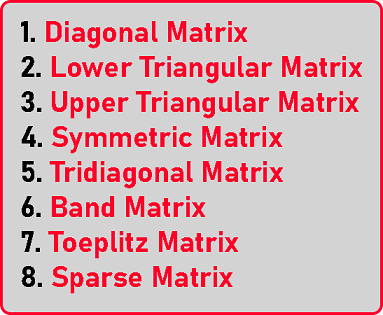# Matrices Introduction

## Matrices Introduction:

In this article, I will give a brief introduction to Matrices and also discuss the Special Matrices. Special Matrices are square matrices i.e. their dimension is n x n. For example, if n is 3 then dimensions will be 3 x 3. It means 3 rows and 3 columns.The matrix should be a square matrix and also it contains more number of 0 elements. If there are more number of 0 elements then we can handle them specially and store only non-zero elements and avoid storage of zero elements. So that space can be saved as well as computation time can be saved. Below is the list of matrices which we will study in coming articles.In the next article, I am going to discuss Diagonal Matrix in C and C++ Language with Examples. Here, in this article, I try to give a brief Introduction to Matrices and I hope you enjoy this Matrices Introduction article.